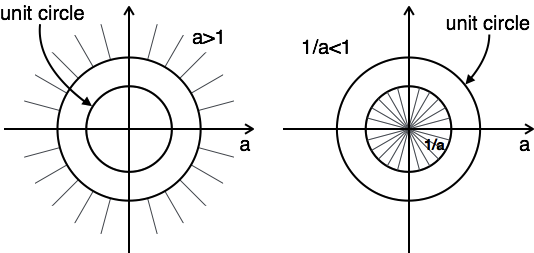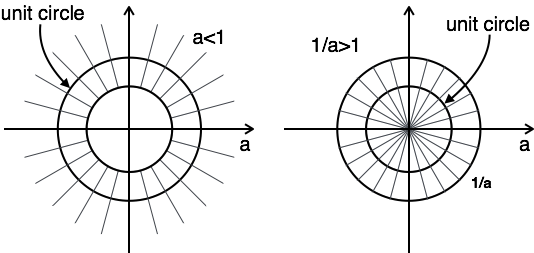# Z-Transforms Properties

Z-Transform has following properties:

### Linearity Property

If $\,x (n) \stackrel{\mathrm{Z.T}}{\longleftrightarrow} X(Z)$

and $\,y(n) \stackrel{\mathrm{Z.T}}{\longleftrightarrow} Y(Z)$

Then linearity property states that

$a\, x (n) + b\, y (n) \stackrel{\mathrm{Z.T}}{\longleftrightarrow} a\, X(Z) + b\, Y(Z)$

### Time Shifting Property

If $\,x (n) \stackrel{\mathrm{Z.T}}{\longleftrightarrow} X(Z)$

Then Time shifting property states that

$x (n-m) \stackrel{\mathrm{Z.T}}{\longleftrightarrow} z^{-m} X(Z)$

### Multiplication by Exponential Sequence Property

If $\,x (n) \stackrel{\mathrm{Z.T}}{\longleftrightarrow} X(Z)$

Then multiplication by an exponential sequence property states that

$a^n\, . x(n) \stackrel{\mathrm{Z.T}}{\longleftrightarrow} X(Z/a)$

### Time Reversal Property

If $\, x (n) \stackrel{\mathrm{Z.T}}{\longleftrightarrow} X(Z)$

Then time reversal property states that

$x (-n) \stackrel{\mathrm{Z.T}}{\longleftrightarrow} X(1/Z)$

### Differentiation in Z-Domain OR Multiplication by n Property

If $\, x (n) \stackrel{\mathrm{Z.T}}{\longleftrightarrow} X(Z)$

Then multiplication by n or differentiation in z-domain property states that

$n^k x (n) \stackrel{\mathrm{Z.T}}{\longleftrightarrow} [-1]^k z^k{d^k X(Z) \over dZ^K}$

### Convolution Property

If $\,x (n) \stackrel{\mathrm{Z.T}}{\longleftrightarrow} X(Z)$

and $\,y(n) \stackrel{\mathrm{Z.T}}{\longleftrightarrow} Y(Z)$

Then convolution property states that

$x(n) * y(n) \stackrel{\mathrm{Z.T}}{\longleftrightarrow} X(Z).Y(Z)$

### Correlation Property

If $\,x (n) \stackrel{\mathrm{Z.T}}{\longleftrightarrow} X(Z)$

and $\,y(n) \stackrel{\mathrm{Z.T}}{\longleftrightarrow} Y(Z)$

Then correlation property states that

$x(n) \otimes y(n) \stackrel{\mathrm{Z.T}}{\longleftrightarrow} X(Z).Y(Z^{-1})$

## Initial Value and Final Value Theorems

Initial value and final value theorems of z-transform are defined for causal signal.

### Initial Value Theorem

For a causal signal x(n), the initial value theorem states that

$x (0) = \lim_{z \to \infty }⁡X(z)$

This is used to find the initial value of the signal without taking inverse z-transform

### Final Value Theorem

For a causal signal x(n), the final value theorem states that

$x ( \infty ) = \lim_{z \to 1} [z-1] ⁡X(z)$

This is used to find the final value of the signal without taking inverse z-transform.

## Region of Convergence (ROC) of Z-Transform

The range of variation of z for which z-transform converges is called region of convergence of z-transform.

### Properties of ROC of Z-Transforms

• ROC of z-transform is indicated with circle in z-plane.

• ROC does not contain any poles.

• If x(n) is a finite duration causal sequence or right sided sequence, then the ROC is entire z-plane except at z = 0.

• If x(n) is a finite duration anti-causal sequence or left sided sequence, then the ROC is entire z-plane except at z = ∞.

• If x(n) is a infinite duration causal sequence, ROC is exterior of the circle with radius a. i.e. |z| > a.

• If x(n) is a infinite duration anti-causal sequence, ROC is interior of the circle with radius a. i.e. |z| < a.

• If x(n) is a finite duration two sided sequence, then the ROC is entire z-plane except at z = 0 & z = ∞.

The concept of ROC can be explained by the following example:

Example 1: Find z-transform and ROC of $a^n u[n] + a^{-}nu[-n-1]$

$Z.T[a^n u[n]] + Z.T[a^{-n}u[-n-1]] = {Z \over Z-a} + {Z \over Z {-1 \over a}}$

$$ROC: |z| \gt a \quad\quad ROC: |z| \lt {1 \over a}$$

The plot of ROC has two conditions as a > 1 and a < 1, as you do not know a.In this case, there is no combination ROC.Here, the combination of ROC is from $a \lt |z| \lt {1 \over a}$

Hence for this problem, z-transform is possible when a < 1.

## Causality and Stability

### Causality condition for discrete time LTI systems is as follows:

A discrete time LTI system is causal when

• ROC is outside the outermost pole.

• In The transfer function H[Z], the order of numerator cannot be grater than the order of denominator.

### Stability Condition for Discrete Time LTI Systems

A discrete time LTI system is stable when

• its system function H[Z] include unit circle |z|=1.

• all poles of the transfer function lay inside the unit circle |z|=1.

## Z-Transform of Basic Signals

x(t)X[Z]
$\delta$1
$u(n)$${Z\over Z-1} u(-n-1)$$ -{Z\over Z-1}$
$\delta(n-m)$$z^{-m} a^n u[n]$${Z \over Z-a}$
$a^n u[-n-1]$$- {Z \over Z-a} n\,a^n u[n]$${aZ \over |Z-a|^2}$
$n\,a^n u[-n-1] $$- {aZ \over |Z-a|^2} a^n \cos \omega n u[n]$${Z^2-aZ \cos \omega \over Z^2-2aZ \cos \omega +a^2}$
$a^n \sin \omega n u[n]$${aZ \sin \omega \over Z^2 -2aZ \cos \omega +a^2 }$

## Useful Video Courses

Video

#### Signals and Systems

239 Lectures 33 hours